# Heat Exchangers (Part - 1) Chemical Engineering Notes | EduRev

## Chemical Engineering : Heat Exchangers (Part - 1) Chemical Engineering Notes | EduRev

The document Heat Exchangers (Part - 1) Chemical Engineering Notes | EduRev is a part of the Chemical Engineering Course Heat Transfer for Engg..
All you need of Chemical Engineering at this link: Chemical Engineering

The Exchanger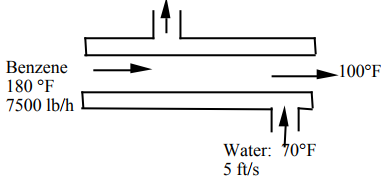The Design Equation for a Heat Exchanger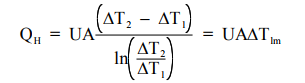Problem : Find the Required Length of a Heat Exchanger with Specified Flows: Turbulent Flow in Both Streams

The design constraints are given in the schematic above. We show this as a countercurrent configuration, but we will examine the cocurrent case as well. The benzene flow is specified as a mass flow rate (in pound mass units), and the water flow is given as a linear velocity. Heat transfer coefficients are not provided; we will have to calculate them based on our earlier discussions and the correlations presented in earlier lectures. The inside tube is specified as "Schedule 40––1-14 inch steel."

Pipe "schedules" are simply agreed-upon standards for pipe construction that specify the wall thickness of the pipe. Perry’s Handbook specifies the following dimensions for

the inside pipe :

Schedule 40 1 1/4” pipe

Do = 1.66 in. = 0.138 ft.

S= πD2 /4 = 0.0104 ft (cross-sectional area for flow)

Di = 1.38 in = 0.115 ft.

the outside pipe :

Schedule 40 2” pipe

Di = 2.07 in = 0.115 ft.

To calculate the heat exchanger area, we must find Ao = πDL. We know the diameter; what is the length ?

The Design Equation is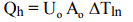The overall heat transfer coefficient, Uo , is given by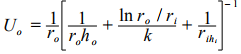We can write it as: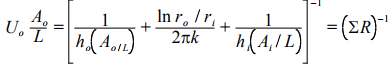To evaluate the parameters of the problem, we need the physical and thermal properties and conditions for flow in the system

Tb = 140˚F,  ρ= 52.3 lbm/ft3 , Cp = 0.45 BTU/lb-°F

kb = 0.085 Btu / h · ft ·°F

μb = 0.39 Cp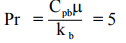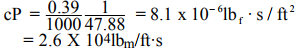Internal Film Resistance

The Nusselt number on the inside of the inner pipe is given by the DittusBoelter equation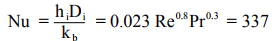so that the film heat transfer coefficient

hi = 249 Btu/h·ft2·˚F

The heat transfer area per unit length is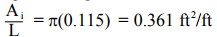so that the inner film resistance is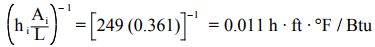The other tube dimensions are

Doi = 0.138 ft and Dio = 0.172 ft

Calculation of the Water Flow Rate

The hydraulic diameter is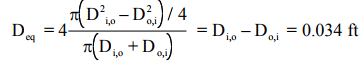Given the water velocity of 5 ft/s, we can solve for the water flow rate

Wwater = 9300 lbm/h

The Overall Energy balance

(wCp ΔT)benz = (wCp ΔT)water

Solving for the outlet water temperature:

7500 (0.45) (100 – 180) = 9300 (1) (70 – Tout)

gives the exit temperature as: Tout = 99˚F

External Film Resistance

The physical properties of the water must be estimated in order to determine the film heat transfer coefficient in the annular shell. The average water temperature Tb is calculated as 84.7 °F

μ = 0.8 cp, k = 0.34 BTU/h-ft-°F , ρ = 62.4 lb/ft3

so that the Reynolds number can be calculated.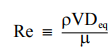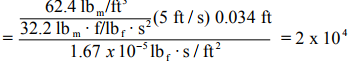From the Dittus-Boelter equation, the Nusselt number is given as:

Nu = 0.023 Re0.8Pr0.4 = 127

so that the external film coefficient, ho , is

ho = 1270 Btu/h·ft2·˚F

The external area/length is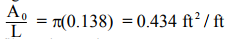so that the external film resistance is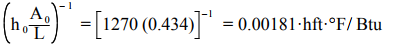Conduction Resistance

The last term in the equation for the overall heat transfer coefficient is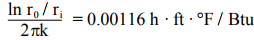Overall Heat Transfer Coefficient

The overall resistance is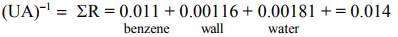Log-Mean ΔT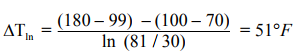Heat Load Qh = wCpΔT = 7500 (0.45) (180 - 100) = 2.7 x 105 Btu/h

Heating Rate/unit Length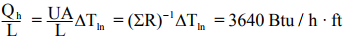Given the heat load, we can calculate the length of tubing so that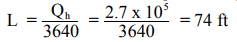The case we considered was countercurrent flow, but we noted in an earlier example that in co-current flow we could be more fluid. Now is the pipe longer or shorter ?

A Co-current Flow Heat Exchanger

The Design Equation for a Heat Exchanger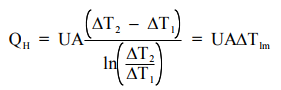The heat loads are identical, the Overall Resistances to heat transfer (UA)-1 are no different since the film coefficients do not change, but the ΔTlm are different.

Counter current

T(water) = 99

T1 (benzene) = 180

T(water) = 70

T2 (benzene) = 100

ΔT= 81

ΔT2 = 30

ΔTlm = 51

L = 74

Co-current

T(water) = 70

T1 (benzene) = 180

T(water) = 99

T2 (benzene) = 100

ΔT= 110

ΔT2 = 1

ΔTlm = 23.2

L = 163 ft

There are two observations to be made. First that the tube length required for co-current flow is more than twice as long. Secondly that the approach temperature for co-current flow becomes diminishingly small.

Offer running on EduRev: Apply code STAYHOME200 to get INR 200 off on our premium plan EduRev Infinity!

## Heat Transfer for Engg.

46 videos|60 docs|59 tests

,

,

,

,

,

,

,

,

,

,

,

,

,

,

,

,

,

,

,

,

,

;